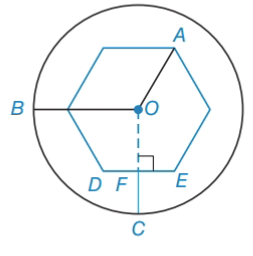Chapter 6.2, Problem 34EElementary Geometry For College St...

7th Edition
Alexander + 2 others
ISBN: 9781337614085

Solutions

Chapter
SectionElementary Geometry For College St...

7th Edition
Alexander + 2 others
ISBN: 9781337614085
Textbook Problem

On a fitting for a hex wrench, the distance from the center o to a vertex is 5 m m The length of radius O B ¯ of the circle is 10 m m . If O C ¯ ⊥ D E ¯ at F , how long is F C ¯ ?To determine

To find:

The length of FC¯.

Explanation

Given:

Calculation:

From the figure,

OA=5OB=10OC¯DE¯

If OFD is a 30060090° triangle.

OD=5DF=2

Still sussing out bartleby?

Check out a sample textbook solution.

See a sample solution

The Solution to Your Study Problems

Bartleby provides explanations to thousands of textbook problems written by our experts, many with advanced degrees!

Get Started

In Problems 21-26, graph each function. 24.

Mathematical Applications for the Management, Life, and Social Sciences

Convert the expressions in Exercises 6584 to power form. 18xx23x35

Finite Mathematics and Applied Calculus (MindTap Course List)

True or False: is a convergent series.

Study Guide for Stewart's Multivariable Calculus, 8th

An integral for the surface area obtained by rotating y = sin x + cos x, , about the x-axis is:

Study Guide for Stewart's Single Variable Calculus: Early Transcendentals, 8th